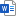# Mathematics

#### Director of Maths

Mrs K Briggs                      K.Briggs@beverleygrammar.co.uk

#### Teachers

Miss J Atkinson                 J.Atkinson@beverleygrammar.co.uk

Dr D Elliott-Button            D.Elliott@beverleygrammar.co.uk

Mr S Knifton                       S.Knifton@beverleygrammar.co.uk

Mrs C Lamplugh               C.Lamplugh@beverleygrammar.co.uk

Mrs A Ryan                         A.Ryan@beverleygrammar.co.uk

### Subject Content

#### KS3

##### Assessment systemMaths Year 7 Assessments structure 2016.docx

##### Year 7 overview
• Calculation and types of number
• Representing discrete data
• Area
• Fractions
• Angle facts
• Percentages
• Expressions
• Simple probability
• Rounding and measures
• Representing continuous data
• Ratio
• Bearings and scale drawing
• Linear equations
• Averages from raw data
• Problem solving.
##### Year 8 overview
• Fractions, decimals and percentages
• Angles and polygons
• Formulae
• Transformations and symmetry
• Combined probability
• Powers and roots
• Straight line graphs
• Circles
• Constructions
• Ratio and proportion
• Sequences
• 3D shapes
• Comparative data
• Compound measures
• Problem solving
• Set 1 only: Pythagoras, Quadratics.
##### Year 9 overview

GCSE Foundation:

• Calculations
• Factors powers and roots
• Angles
• Fractions decimals and percentages
• Averages
• Expressions
• Constructions and 3D drawing
• Ratio
• Sequences
• Transformations and scale drawings
• Compound measures
• Simple probability
• Rounding and bounds
• Area
• Problem solving

GCSE Higher:

• Calculations
• Ratio
• Factors primes and multiples
• Angles
• Fractions decimals and percentages
• Expressions and linear equations
• Averages
• Constructions
• Sequences
• Transformations
• Compound measures
• Simple probability
• Rounding and bounds
• Coordinate geometry of straight lines
• Handling data
• Proportionality
• Problem solving

#### KS4### Useful websites

MyMaths – www.mymaths.co.uk

Kerboodle (Online textbook) – www.kerboodle.co.uk

Exam board – www.aqa.org.uk